#Interactive Real Analysis

Next | Previous | Glossary | Map

## 7. The Integral

### 7.1. Riemann Integral

In a calculus class integration is introduced as 'finding the area under a curve'. While this interpretation is certainly useful, we instead want to think of 'integration' as more sophisticated form of summation. Geometric considerations, in our situation, will not be so fruitful, whereas the summation interpretation of integration will make many of its properties easy to remember.

First, as usual, we need to define integration before we can discuss its properties. We will start with defining the Riemann integral and we will move to the more technical but also more flexible Lebesgue integral later.

 Definition 7.1.1: Partition of an Interval A partition P of the closed interval [a, b] is a finite set of points P = { x0, x1, x2, ..., xn} such that a = x0 < x1 < x2 < ... < xn-1 < xn = b The maximum difference between any two consecutive points of the partition is called the norm or mesh of the partition and denoted as | P |, i.e. | P | = max { xj - xj-1, j = 1 ... n } A refinement of the partition P is another partition P' that contains all the points from P and some additional points, again sorted by order of magnitude.
 Examples 7.1.2:What is the norm of a partition of 10 equally spaced subintervals in the interval [0, 2] ?What is the norm of a partition of n equally spaced subintervals in the interval [a, b] ?Show that if P' is a refinement of P then | P' || P |.
Using these partitions, we can define the following finite sum:
 Definition 7.1.3: Riemann Sums If P = { x0, x1, x2, ..., xn} is a partition of the closed interval [a, b] and f is a function defined on that interval, then the n-th Riemann Sum of f with respect to the partition P is defined as: R(f, P) =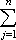f(tj) (xj - xj-1) where tj is an arbitrary number in the interval [xj-1, xj].Note: If the function f is positive, a Riemann Sum geometrically corresponds to a summation of areas of rectangles with length xj - xj-1 and height f(tj).

 Examples 7.1.4:Suppose f(x) = x2 on [0, 2]. Find the fifth Riemann sum for an equally spaced partition, taking always the left endpoint of each subinterval the fifth Riemann sum for an equally spaced partition, taking always the right endpoint of each subinterval the n-th Riemann sum for an equally spaced partition, taking always the right endpoint of each subinterval.
Riemann sums have the practical disadvantage that we do not know which point to take inside each subinterval. To remedy that one could agree to always take the left endpoint (resulting in what is called the left Riemann sum) or always the right one (resulting in the right Riemann sum). However, it will turn out to be more useful to single out two other close cousins of Riemann sums:
 Definition 7.1.5: Upper and Lower Sum Let P = { x0, x1, x2, ..., xn} be a partition of the closed interval [a, b] and f a bounded function defined on that interval. Then: the upper sum of f with respect to the partition P is defined as: U(f, P) =cj (xj - xj-1) where cj is the supremum of f(x) in the interval [xj-1, xj]. the lower sum of f with respect to the partition P is defined as L(f, P) =dj (xj - xj-1) where dj is the infimum of f(x) in the interval [xj-1, xj].
 Here is an example where the upper sum in displayed in dark brown and the lower sum in light brown. The partition P = {0.5, 1, 1.5, 2}, and the numbers for the sums are chosen: for the upper sum: c1 = f(1), c2 = f(1.5), and c3 = f(1.5) for the lower sum: d1 = f(0.5), d2 = f(1), and d3 = f(2)Examples 7.1.6:Suppose f(x) = x2-1 for x in the interval [-1, 1]. Find: The left and right sums where the interval [-1, 1] is subdivided into 10 equally spaced subintervals. The upper and lower sums where the interval [-1, 1] is subdivided into 10 equally spaced subintervals. The upper and lower sums where the interval [-1,1] is subdivided into n equally spaced subintervals.Why is, in general, an upper (or lower) sum not a special case of a Riemann sum ? Find a condition for a function f so that the upper and lower sums are actually special cases of Riemann sums.Find conditions for a function so that the upper sum can be computed by always taking the left endpoint of each subinterval of the partition, or conditions for always being able to take the right endpoints.Suppose f is the Dirichlet function, i.e. the function that is equal to 1 for every rational number and 0 for every irrational number. Find the upper and lower sums over the interval [0, 1] for an arbitrary partition.
These various sums are related via a basic inequality, and they are also related to a refinement of the partition in the following theorem:
 Proposition 7.1.7: Size of Riemann Sums Suppose P = { x0, x1, x2, ..., xn} is a partition of the closed interval [a, b], f a bounded function defined on that interval. Then we have: The lower sum is increasing with respect to refinements of partitions, i.e. L(f, P')L(f, P) for every refinement P' of the partition P The upper sum is decreasing with respect to refinements of partitions, i.e. U(f, P')U(f,P) for every refinement P' of the partition P L(f, P)R(f, P)U(f, P) for every partition P

In other words, the lower sum is always less than or equal to the upper sum, and the upper sum is decreasing with respect to a refinement of the partition while the lower sum is increasing with respect to a refinement of the partition. Hence, a natural question is: will the two quantities ever coincide ?

 Definition 7.1.8: The Riemann Integral Suppose f is a bounded function defined on a closed, bounded interval [a, b]. Define the upper and lower Riemann integrals, respectively, as I*(f) = inf{ U(f,P): P a partition of [a, b]} I*(f) = sup{ L(f,P): P a partition of [a, b]} Then if I*(f) = I*(f) the function f is called Riemann integrable and the Riemann integral of f over the interval [a, b] is denoted byf(x) dx
Note that upper and lower sums depend on the particular partition chosen, while the upper and lower integrals are independent of partitions. However, this definition is very difficult for practical applications, since we need to find the sup and inf over any partition.
 Examples 7.1.9:Show that the constant function f(x) = c is Riemann integrable on any interval [a, b] and find the value of the integral.Is the function f(x) = x2 Riemann integrable on the interval [0,1] ? If so, find the value of the Riemann integral. Do the same for the interval [-1, 1].Is the Dirichlet function Riemann integrable on the interval [0, 1] ?
The third example shows that not every function is Riemann integrable, and the second one shows that we need an easier condition to determine integrability of a given function. The next lemma provides such a condition for integrability.
 Lemma 7.1.10: Riemann Lemma Suppose f is a bounded function defined on the closed, bounded interval [a, b]. Then f is Riemann integrable if and only if for every> 0 there exists at least one partition P such that | U(f,P) - L(f,P) | <Examples 7.1.11:Is the function f(x) = x2 Riemann integrable on the interval [0,1] ? If so, find the value of the Riemann integral. Do the same for the interval [-1, 1] (since this is the same example as before, using Riemann's Lemma will hopefully simplify the solution).Suppose f is Riemann integrable over an interval [-a, a] and { Pn } is a sequence of partitions whose mesh converges to zero. Show that for any Riemann sum we have lim R(f, Pn) =f(x) dxSuppose f is Riemann integrable over an interval [-a, a] and f is an odd function, i.e. f(-x) = -f(x). Show that the integral of f over [-a, a] is zero. What can you say if f is an even function?
Now we can state some easy conditions that the Riemann integral satisfies. All of them are easy to memorize if one thinks of the Riemann integral as a somewhat glorified summation.
 Proposition 7.1.12: Properties of the Riemann Integral Suppose f and g are Riemann integrable functions defined on [a, b]. Thenc f(x) + d g(x) dx = cf(x) dx + dg(x) dx If a < c < b thenf(x) dx =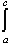f(x) dx +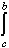f(x) dx |f(x) dx || f(x) | dx If g is another function defined on [a, b] such that g(x) < f(x) on [a, b], theng(x) dxf(x) dx If g is another Riemann integrable function on [a, b] then f(x) . g(x) is integrable on [a, b]
 Examples 7.1.13:Find an upper and lower estimate forx sin(x) dx over the interval [0, 4].Suppose f(x) = x2 if x1 and f(x) = 3 if x > 1. Findf(x) dx over the interval [-1, 2].If f is an integrable function defined on [a, b] which is bounded by M on that interval, prove that M (a - b)f(x) dxM (b - a)
Now we can illustrate the relation between Riemann integrable and continuous functions.
 Theorem 7.1.14: Riemann Integrals of Continuous Functions Every continuous function on a closed, bounded interval is Riemann integrable. The converse is false.

Note that this theorem does not say anything about the actual value of the Riemann integral. Also, we have as a free extra condition that that f is bounded, since every continuous function on a compact set is automatically bounded.

Since differentiable functions are continuous, this result implies that

{ differentiable functions }{ continuous functions }{ integrable functions }
and each set is in fact a proper subset of the next.
 Examples 7.1.15:Find a function that is not integrable, a function that is integrable but not continuous, and a function that is continuous but not differentiable.
To finalize the relation between integrable and continuous functions, the following theorem can be proved (but it uses the concept of a measure, defined later):
 Theorem 7.1.16: Lebesgue's Theorem If f is a bounded function defined on a closed, bounded interval [a, b] then f is Riemann integrable if and only if the set of points where f is discontinuous has measure zero.
A set of measure zero, as we will see later, is any set of finitely or countably many points. Therefore, we could rephrase the above exact theorem as follows:
 Corollary 7.1.17: Riemann Integral of almost Continuous Function If f is a bounded function defined on a closed, bounded interval [a, b] and f is continuous except for at most countably many points, then f is Riemann integrable.

The converse is not quite true: If f is a bounded function defined on a closed, bounded interval [a, b] and f is Riemann integrable, then f is continuous on [a, b] except possibly at a set of measure zero, but a set of measure zero does not necessarily consist of countably many points.

 Examples 7.1.18:Show that every monotone function defined on [a, b] is Riemann integrable.Let g(x) = 0 if x is irrational and g(p/q) = 1/q if x = p/q is rational, p, q relatively prime and q > 0, and assume g is restricted to [0, 1]. Is g Riemann integrable ? If so, what is the value of the integral ?
Now that we have easy conditions to determine which functions are integrable, it would also be convenient to have a nice shortcut to easily compute the actual value of an integral.
 Theorem 7.1.19: Fundamental Theorem of Calculus Suppose f is a bounded, integrable function defined on the closed, bounded interval [a, b], define a new function: F(x) =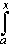f(t) dt Then F is continuous in [a, b]. Moreover, if f is also continuous, then F is differentiable in (a, b) and F'(x) = f(x) for all x in (a, b) In many calculus texts this theorem is called the Second Fundamental Theorem of Calculus.
This theorem has an easy corollary that enables us to quickly find the value of an integral in many situations.
 Corollary 7.1.20: Integral Evaluation Shortcut Suppose f is an continuous function defined on the closed, bounded interval [a, b], and G is a function on [a, b] such that G'(x) = f(x) for all x in (a, b). Thenf(x) dx = G(b) - G(a) The function G is often called Antiderivative of f, and this corollary is called First Fundamental Theorem of Calculus
Before we look at several examples, we should rephrase these results in less rigorous notation. The first theorem says, basically: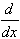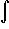f(x) dx = f(x)
while the corollary states, basically, that:f(x) dx = f(b) - f(a)
Hence, loosely speaking, integration and differentiation are inverse operations of each other.
 Examples 7.1.21:Define a function F(x) =t2 sin(t) dt for x in the interval [a, a + 10]. Find F(a) Find F'(x) Find F''(x) Find all critical points of F(x) in [a, a + 10]Find the value of the following integrals:x5 - 4 x2 dx on the interval [0, 2].1/x2 + cos(x) dx on the interval [1, 4].(1 + x2)-1 dx on the interval [-1, 1].Show that if one starts with an integrable function f in the Fundamental Theorem of Calculus that is not continuous, the corresponding function F may not be differentiable.
In the next chapter we will learn some more shortcuts to compute the value of an integral called substitution method and integration by parts.
Next | Previous | Glossary | Map